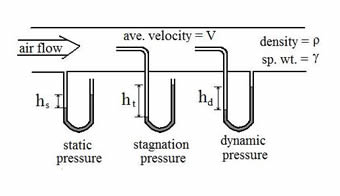# Difference between Static and Dynamic Pressure

##### Key Difference: Dynamic pressure is the continuous physical force exerted on an object by something such as air on fluid. Static pressure is the physical force exerted on an object that is not in motion.Dynamic and static pressure are used in fluid dynamics to under the pressure that is exerted on an object. These terms are a part of Bernoulli’s principle are used as an energy balance on a closed system. The three terms are used to define the state of a closed system of an incompressible, constant-density fluid.

Let’s simply to understand this concept. It is quite a simple concept that requires little explanation. Static and dynamic pressure are often used to determine the pressure in a closed system. So assuming that we are talking about a fluid (water, mist, etc.) in a pipe. This piece of pipe is placed horizontally and is filled with liquid.The liquid inside this pipe is stationary and not moving, but that doesn’t mean that there is no pressure on it. The air provides pressure pushing it downwards, this is known as the static pressure when the liquid is at a state of rest. Now, imagine there is rushing water in the pipe, the pressure put placed by the water pushing out against the present liquid, this is known as dynamic pressure.

This is commonly the only difference. The pressure placed when there is no movement is static pressure, while pressure because of movement is dynamic pressure.

Comparison between Dynamic and Static Pressure:

 Dynamic Pressure Static Pressure Definition Is the continuous physical force exerted on an object by something such as air on fluid The physical force exerted on an object that is not in motion Field Fluid dynamics Fluid dynamics, design and operation of an aircraft and fluid statistics Motion The object is motion The object is stagnant

Image Courtesy: forum.grasscity.com, brighthubengineering.com